4. Equilibrium

Content
4.1. General
4.3. Calculation of a Thermodynamic Equilibrium

4.1. General

The application calculates the thermodynamic equilibrium at the given temperature and pressure or volume for isothermal or adiabatic conditions.
By variation of the system conditions e.g. temperature, pressure and mass of substances, the results of several calculations can be shown as table and graphic in depend of the variant parameters.
The number of phases can be up to 8 (2 gas, 3 liquid and 3 solid). The maximum number of substances each phase is 255 (allowing 2040 substances in total).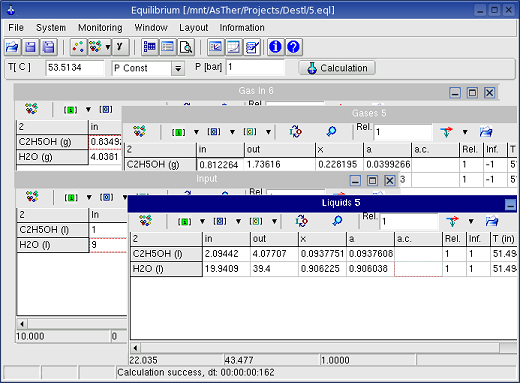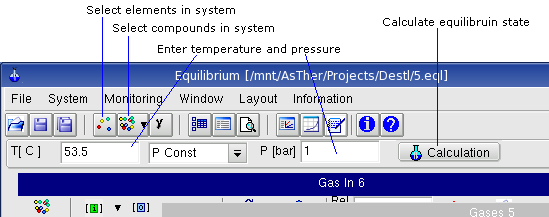File -> Close deselects defined system (elements and compounds)
File -> Exit: closes the application.

System -> Elements shows the following dialog box, in which the elements of the system can be selected. When the buttons for the elements are pressed, the colours will be changed to red or blue. The buttons of the selected elements are shown in red text.  When electron is selected, ions can be involved for the calculation.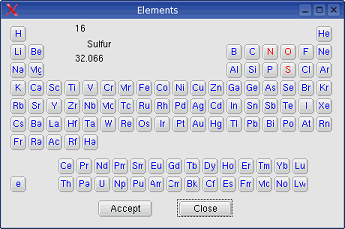System -> New phase:
As default three phases are given from the start (gas, liquid and solid).
System -> New phase -> Gas: An additions gas phase will be created if the number of present phases is less than two.
System -> New phase -> Liquid: An additional liquid phase will be created if the number of present phases is less than three.
System -> New phase -> Solid: An additional solid phase will be created if the number of present phases is less than three.

System -> Compounds: When the elements are selected, the substances from these elements can be selected. According to the choice of elements the substances available in the database are displayed in an dialog box.In this dialog box,  the title of the phase windows can be changed. The drop-down-box Containing enables to constrict the shown substances in the Database-Box
In the picture above, when N is selected in the drop down box, only substances will be shown in the Database-Box, which contains N, and which is consisting from C, H, O, N, S: corresponding to the element selection.

System -> Behaviour: You can select, according to what law the gases should be treated. The state functions can be calculated according to the law of real gases, when the critical data (Tc, Pc) of the gases are known.System -> Calculation Options: Shows a dialog box for the following settings: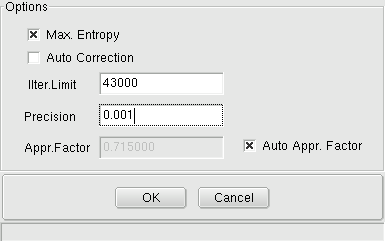Max. Entropy  when it is checked,  it is not necessary to include all substances of the real system, i.e. if the real system comprises e.g.: Ag (l), H2O (g), H2 (g), O2 (g) H2S (g) and sulphur solved in silver, the calculation can be executed without Ag(l), if Ag(l) is not participating in the reaction. It recommended, that Max Entropy Box should be checked, because all but impossible, that all the really existing substances to include in a calculation.

Auto correction, when it is checked, the application Equilibrium inspects, whether expected pure substances (to be defined as such by setting the activity-coefficient to (1) can be formed in the given system. If the formation of such pure substances is impossible, the calculation will be repeated automatically without considering the generation of this substance. When auto correction is checked, you should always look at the message window, if the calculation can be carried out without errors.

System-> Environment -> Isotherm / Adiabatic (alternate menu): When adiabatic is selected,  the temperature will be calculated and displayed in the input field for the temperature. Your input in this field will be taken as a starting value for the calculation. After calculation, this value will be overwritten by the calculation result.

System-> Calculation: carries out a calculation.

System -> Calculation by Variation shows a dialog box, you can enter initial values of the mass or temperature, pressure as well as volume as variable, so that several calculations will be carried out corresponding to given parameter. This option is especially useful for the tabular and graphical display of calculation results .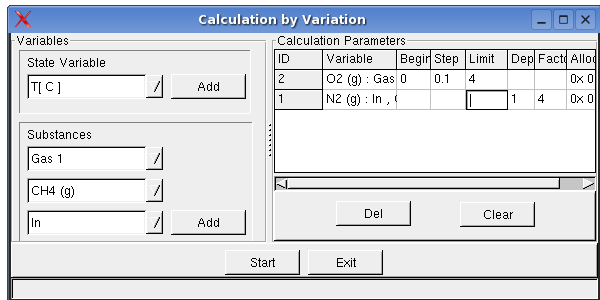Variables can be added by the Buttons Add. The values can be entered in the Begin-, End- and Step-Columns. The column Dependence can be used optional. The ID of a variable above  can be given. The Factor is necessary, when a dependence ID is given. The column Allocation is set and used by the application. Users can not enter any data

System->Activity coefficient: Dialog box to define activity coefficients as functions of concentration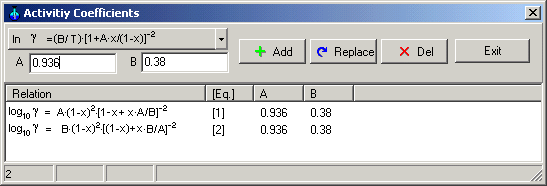Equations for activity coefficients can be select using drag down button.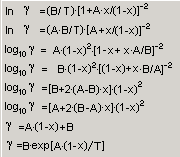When the Button Add is pressed, the ID of equations ( , , , ...) will be given by the application in the dialog box. When this number is referred to in the f.c.- or a.c.- columns in the windows of a phase, the activity coefficients will be calculated according to the chosen relations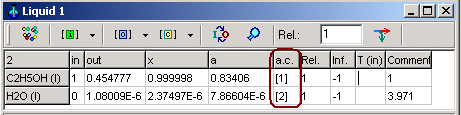Important rules:
1.  when an equation is used for activity coefficient of a substance, which is depend on the concentration, you should repeat the calculation at given T, P or V,  until the calculated concentration is changed only in a negligible range. Because, the application sets as initial value of the activity coefficient corresponding to the values in the concentration column (usually x , mol ratio)

2. You sould always set the concentration in mol ration x, not weight ratio or weight %. When you define the activity coefficient by an equation and the concentration is selected other than mol ratio x, application shows an error message and breaks the calculation

Monitoring -> Record Variables shows a dialog box, in which the record variables can be selected.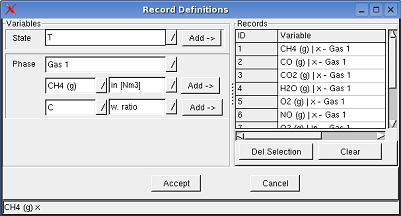When a variable is selected to record, the entered and/or calculated values of the variables written in an table, when a calculation is executed.

Monitoring -> Record Table shows the record table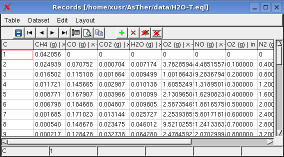Monitoring -> Elemental Balance, The distribution of the elements in substances before and after the equilibrium is displayed tabular in a window.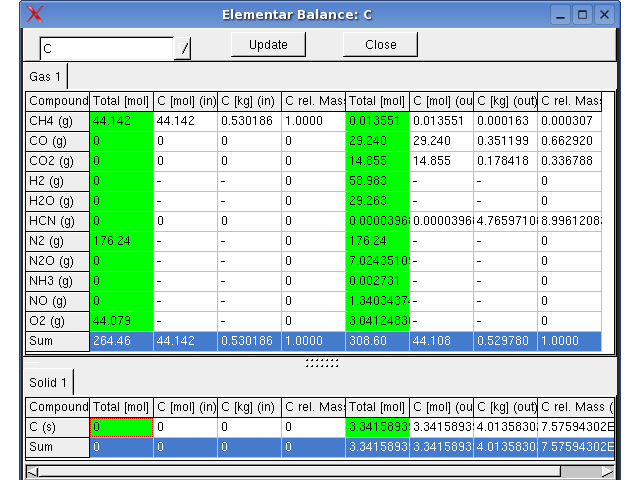4.3. Toolbar

The compounds of the phases, the dimensions in the input-, output- and concentration-columns can be set using the toolbar buttons.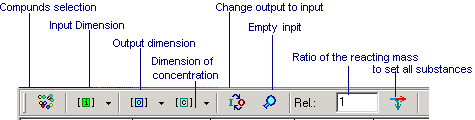If  [mol] is selected as dimension of the input data (default settings), the actual input values can be given explicitly with another dimension during the input procedure as for example: 1 kg, 2 lb, 51,6 m3, 7 Nm3. If no specific dimension is given during input, mol will be taken as dimension. If other dimensions are selected, any dissimilar given dimension during input will produce an error message.

4.4. Popup Menus of the Windows for Phases
When the right mouse button is pressed in a window for phases, a pop up menu will be shown: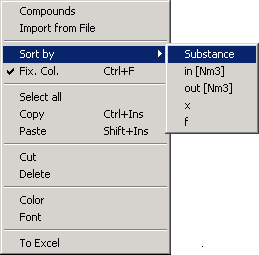Compounds: a dialog box will be shown, in which the compounds of the phase can be selected
Sort by
: the data will be sorted according to selected criteria
To Excel: transfers the table to an MS Excel Application (MS Windows only)

4.5. Calculation of a Thermodynamic Equilibrium
4.5.1. Simple calculation

4.3.1.1. Select the elements in thermodynamic system: menu System -> Elements.
4.3.1.2. Select the compound of the existing phases, Press button comp. in each windows
4.3.1.3. Enter initial mass values in the tables into the in-column of the table in the phase windows. I
4.3.1.4. Start the Calculation: Press Button Calculation

If the calculation is carried out under constant volume, the selected volume refers to the equilibrium state after the reaction (out).
The state functions of the input species will be calculated from the given settings under a pressure of 1 bar.
The gaseous products will be calculated under the pressure that results from the reaction.

4.5.2. Phases consisting of pure substances or mixtures
The application does not make a decision for coexistence of compounds in the same phase as mixture. When a substance can only exists as a pure substance in a separately phase, you must set (1) in the a.c.-columns. In other cases, all substances are regarded as compounds of a mixture

The following example shows for different mixing effects of C(s), S(s) and S(l), when they are defined as pure substances setting (1) for activity coefficients.

 C (s) and S (s) are within a mixture C(s), S(s) and S(l) are formed as pure substances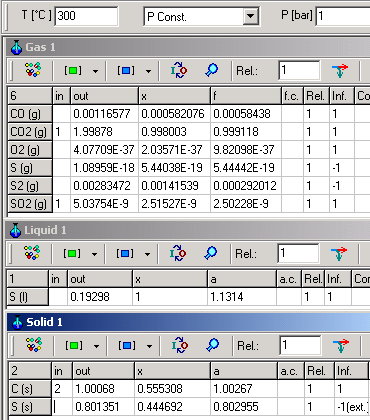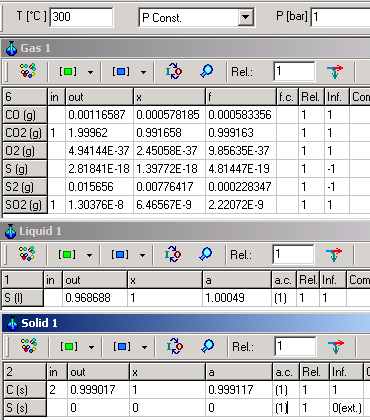For both cases, following equation is valid:
C (s) + SO2 (g) = S (l) + CO2 (g)   K=e-ΔG�/RT= 4.5 . 108

When C(s) and S(l) are in mixture, K can be calculated as:
K= aS(l).PCO2/(aC(s).PSO2)=1.1314 . 0.999118/(1.00267 . 2.50228 10-9) = 4.5 . 108

If, however, C(s) and S(l) are pure substances in separately phases K will be calculated as:
K= PCO2/PSO2=0.999163/2.22072 10-9 = 4.5 . 108

Although different in result, both calculations are consistently calculated according to thermodynamic rules.

4.5.3. Results of the Calculation

4.5.3.1. Info-Column
-1: The formation is inhibited
0:  The formation is inhibited, the substance can not exists as pure substance, the substance can exists only in a mixture
1:  Formation is possible.
The possibility of the formation of the species are always relative with respect to the thermodynamic system and its inherent components. In a systems containing CO2(g), CO(g), H2(g), O2(g) and C(s) of O2(g),  when there are O2- excess, the formation of C(s) (pure solid carbon) is inhibited. Therefore it info-column value will be set to -1 after the calculation.
If, however, the system also contains CH4(g), the  formation of C(s) possible,  In the info-column, the result 1 my be written.

4.5.3.2. All Substances are considered in mixtures if not otherwise specified
In a system containing FeO(s), Fe(l), Ca(l), CaO(s) at 1200 �C and 1 bar, Fe (l)-mass will have an output value > 0 and the plausibility value will be 1 as long as there are other liquid components allowing for mixture. If, however, there should be no Ca(l) (for example) allowed, the output of Fe(l) will be 0 because no liquid mixture is possible. Accordingly the plausibility value will be set to 0. In the first case, you will have to decide, whether a mixture of Fe(l) and Ca(l) is possible. If you see 0 or -1 in the info-column after the calculation, when the coexistence Fe(l) and Ca(l) is not possible in a mixture, this substances should be deselected from this phase. The program itself takes no decision, if any mixture is possible or, if the coexistence of the substances in a mixture is possible.

4.5.3.3. Selection of compounds
The algorithms of the equilibrium calculations in AsTher differs from other algorithms. In AsTher, there is no need to include elementary substances for reference reasons. In a system consisting from CH4, CO2, CO, O2, N2 and NO, when O2(g) exists more than enough to the formation of  CO2 und H2O, C(s) and H2(g) have not to be selected in order to create plausible results. This is no longer necessary in Equilibrium.

4.5.3.4. Graphic Window
The result of the calculation can be recorded in a table. Which variables are to record, must be defined.
When calculation variables are recorded, the data of the record table can be shown in a graphic window.
You can define, which recorded variables are to show in the graphic window, menu: Monitoring -> Graphic Variables
Monitoring -> Graphic: shows the graphic window.
More About Graphic Windows in Cap.7.

4.6. Some Tips for the User Interface
A calculation can be break, if no equilibrium can be reached under the given number of maximum iteration steps and precision.
In this case, the iteration settings have to be altered.

In order to create a better overview, the phase windows can be minimised. Those phase windows holding no components can be closed.

The width and height of tables can be changed as shown in the picture below.

4.7. Reliablity of the Calculations
4.7.1. In general, several calculations should be carried out in different temperatures in the expected range in the real system. It is very rarely, that in an real  system a homogenous temperature distribution. You should compare the result of the calculation in the graphic- or table-window.

4.7.2. The given precision value might occasionally have an high influence on the calculation result. Therefore it is advisable to repeat the calculation with another precision and compare the result. If there should be a considerable difference in the results, there might be limiting or faulty preconditions given in the system, which force AsTher to calculate wrong results.

4.8. Errors and Trouble shooting

4.8.1. If the mass-conservation is not possible, the calculation will be terminated. If, for example, a system comprising the elements H and O in the substances H2(g) and H2O(l), but no Oxygen in the products, the calculation under 1 bar and 1000 K will stop. To avoid this, it is recommended to incorporate all potential product substances in the first step and erase those which will not be created in a second step.
4.8.2. Error messages related to operations system faults may occur due to excessively high values of the free energy of a substance, so that the processor can not interpret the value. In this case, please check the thermodynamic data set.

4.8.3. Two reaction systems with high different quantities may produce erroneous results.
If, for example, a system consisting of Fe, Cl2, FeCl2, H2O,CO,CO2,N2,NO ,
wherein one reaction system is consisting of Fe-Cl2-FeCl2 and  the other consisting of C-CO-H2O-N2-NO.
If the inputs of the calculation is 10000 mol FeCl2, 1 mol H2O and 1 mol N2, 0.1 mol C, and the precision is 0.01,
the calculation result my be incorrect for the system C-CO-H2O-N2-NO.
It is recommended to calculate in separate systems.

4.8.4. Pure Substances :
If a substance my be exists as a pure substance (and accordingly the activity coefficient has been set to (1) in the a.c./f.c.- column, please check after the calculation, whether:
- the info column shows the value 1,
- the amount of that substance is more than 1e-23 mol,
- the calculated value or the activity is nearly 1.
If not, those substances should be excluded from the calculation, e.g. by setting the a.c.-value to 0. This can also be done automatically by AsTher using Menu: System-> Extended Options, in the following dialog box, check the option  auto correction, which, however, causes longer calculation time.

4.8.5. An Obviously Erroneous Result (e.g. the formation of liquid Fe at 20 �C), although the result are thermodynamically consistent. This may happen, if the extrapolation is enabled and the extrapolated data itself are inconsistent. With the application Pure Substance, you can follow the course of the free Enthalpy in graphic and table window in order to check the validity range of the required dataset.

4.8.6. Erroneous calculation can be caused by the incomplete definition of the system. The system can be influenced by mass of the gaseous substances, although these substance can not take in any reaction. The results of the calculation will be is different in a system consisting of CaO, CaCO3, CO and N2, when the mass of N2 is different, although N2 does not react..

4.8.7. Optimization of the Iteration
A calculation will be terminated if there is no solution to be found within the predefined number of iteration steps which would suit the desired precision.
In this case, please check first, if any message is shown,
When no another error message is shown, the calculation can be successfully carried out, if
- the number of iteration steps is increased or
- the required precision (-value) is increased
permitted range and (default) values:
Range of iteration steps: 1 - 2 147 483 647 (43 000)
Range of precision (value): (>0) - 0.1